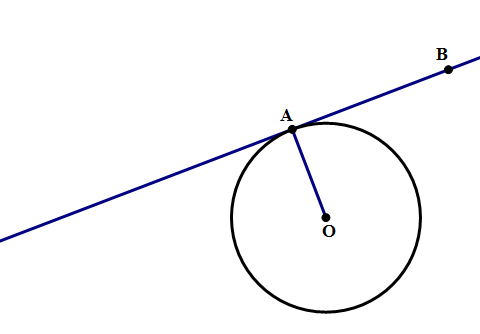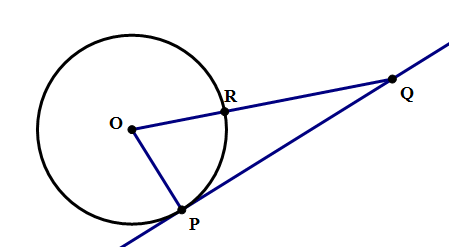# Math: Circle and Line Diagrams

A series of five articles on circles.

What happens when a straight line comes near a circle? In how many ways can these two objects intersect? It turns out, there are three possibilities — see below: no intersection, one intersection, or two intersections.

In the first case, no intersection, nothing interesting happens, so there’s no special name for that. In the second case, the line just “touches” circle at one point, so it’s called a tangent line; the word tangent, a “touching” line, shares an etymological root with the word tangible, meaning “touchable.” In the third case, the line cuts through the circle, so it’s called a secant line; the word secant means “cutting”, and shares an etymological root with other “cutting” words, such as: dissection, intersection, bisect, sector, section, etc.

There’s nothing particular special about secant lines. The part of the secant line between the two intersection points is, of course, a chord. Remember, the diameter is a chord, the longest possible chord, so the chord on a secant line would be diameter if the secant line happened to pass through the center of the circle. In Geometry, there are sorts of complicated theorems about the angle between two secant lines both intersecting the same circle, but that gets into territory well beyond the Quant.

## Tangent lines

Tangent lines are more interesting, and there’s a very important fact about tangent line that the QUANT will expect you to know. Consider a line tangent to a circle at point \space A, and a radius also drawn to point \space A.

As you might suspect: a tangent line is always perpendicular to a radius at the same point. In other words, it absolutely must be true that \angle{OAB} is exactly a 90\degree angle. That’s a fact you need to know for the Quant section.

## Practice problems1) In the diagram above, line \space PQ is tangent to the circle, and the measure of arc PR is 70\degree. What is the measure of \angle{PQR}?

A. 15\degree

B. 20\degree

C. 25\degree

D. 30\degree

E. 35\degree2) In the diagram above, lines \space EF and DE are each tangent to the circle. If \angle{FOD} = 110\degree, what is the measure of \angle{DEF}?

A. 40\degree

B. 50\degree

C. 60\degree

D. 70\degree

E. 80\degree

## Practice problem explanations

1) First of all, since the measure of arc PR is 70\degree, we know the angle at the center, \angle{POR}, must also be 70\degree. We know that \angle{OPQ} = 90\degree, because PQ is a tangent line. Now look at \triangle{OPQ}. We know that the three angles in that triangle must add up to 180\degree.

\angle{POR} + \angle{OPQ} + \angle{PQR} = 180\degree

70\degree + 90\degree + \angle{PQR} = 180\degree

160\degree + \angle{PQR} = 180\degree

\angle{PQR} = 180\degree - 160\degree = 20\degree